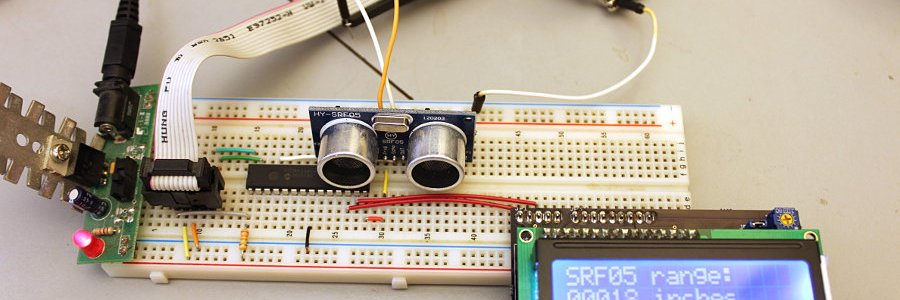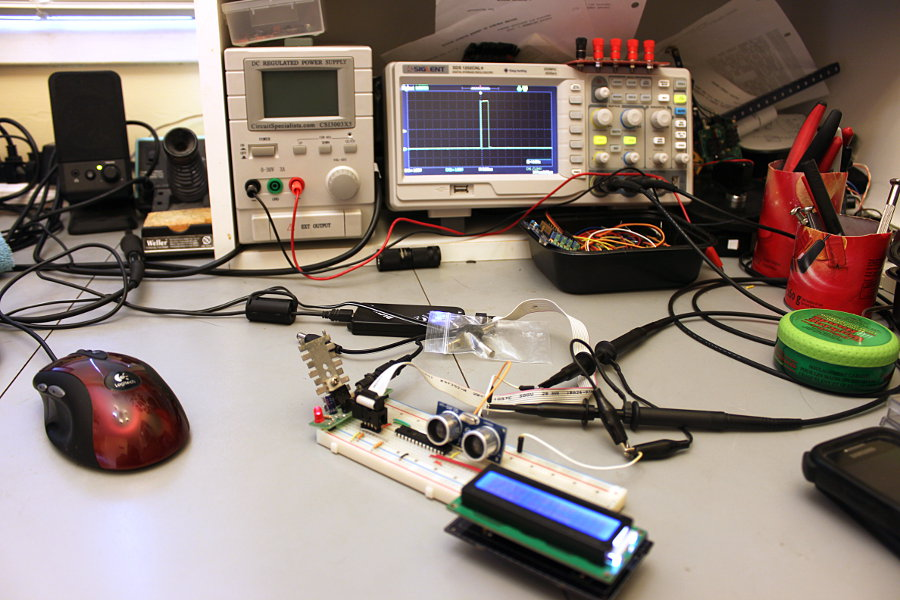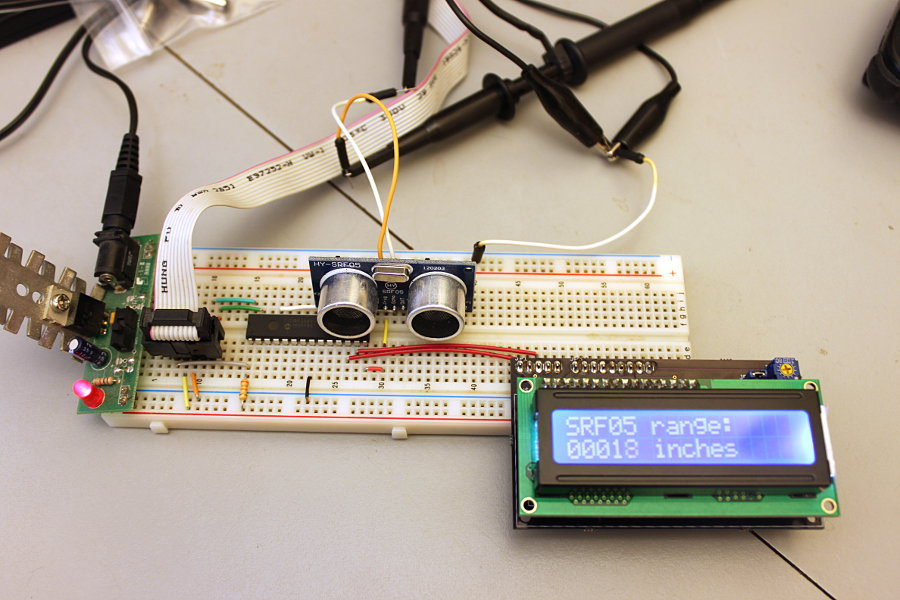#### HY-SRF05 Ultrasonic RangefinderIn a recent Ebay China toy order I grabbed four HY-SRF05 ultrasonic rangefinder sensors to play with. Can't beat the price at \$1.89 USD each with free shipping. They'd be dirt cheap at twice that price.

Here I'm controlling the SRF05 with a PIC18F2680 and displaying the results on my "Predko" 2-wire 16x2 LCD. The scope is triggering on the start of the SRF05 measurement pulse so I can watch the pulse width change as the SRF05 measures different distances.This is a nice easy to use, and very accurate sensor! It does get some bogus readings sometimes, like any rangefinding sensor, but most of the time it's very good.At first I was having trouble with my interrupt code, so I wrote this super simple program to activate and read the sensor without interrupts. It works fine, but won't be useful for much except as a demo.

I know my lcd_16number() function isn't very good. It's a thing I wrote long ago, before I learned better ways of doing that. I just quickly found it and plugged it in here - couldn't find my better function and was too lazy to write one. It should be replaced with something more efficient and elegant for sure.

(scroll way down for working interrupt code)

``````#include <system.h>

#pragma DATA    _CONFIG1H, _OSC_HS_1H
#pragma DATA    _CONFIG2H, _WDT_OFF_2H
#pragma DATA    _CONFIG3H, _MCLRE_ON_3H
#pragma DATA    _CONFIG4L, _LVP_OFF_4L & _XINST_OFF_4L
#pragma CLOCK_FREQ 20000000

//function prototypes
void lcd_cls();
void lcd_string(char *);
void lcd_cmd(unsigned char);
void lcd_char(char);
void e_togg(void);
void lcd_init(void);
void lcd_nybble(unsigned char,unsigned char);
void lcd_16number(int);

//LCD defines
#define line1 lcd_cmd(0x80);
#define line2 lcd_cmd(0xc0);
#define CLOCK latc.1
#define DATA  latc.2

//SRF05 defines
#define TRIG  latb.0
#define ECHO  portb.4

unsigned int time = 0;
bit flag = 0;
char string[] = "                ";
char number[] = "      inches    ";

void main()
{
osccon = 0b01110000;    //20MHz external osc
trisa = trisc = 0;
latc = 0;
trisb = 0b00010000;     //RB4 is ECHO input
TRIG = 0;
t1con = 0b00110000;     //set up Timer1, 1:8, not enabled

lcd_init();
lcd_string("SRF05 range");
delay_s(1);

while(1){
tmr1h = 0;                    //zero Timer1
tmr1l = 0;

TRIG = 1;                     //activate SRF05
delay_10us(1);
TRIG = 0;

while(ECHO == 0){             //wait for pulse start
}

t1con.TMR1ON = 1;             //enable Timer1

while(ECHO == 1){             //wait for pulse end
}

t1con.TMR1ON = 0;             //stop Timer1
time = time / 37;             //convert to inches
lcd_16number(time);           //convert Timer1 to ASCII
line2;
lcd_string(number);           //and display it
delay_ms(100);
}
}

void lcd_16number(int num)
{
number=(char)(abs(num/10000)+0x30);
num=num-abs(num/10000)*10000;
number=(char)(abs(num/1000)+0x30);
num=num-abs(num/1000)*1000;
number=(char)(abs(num/100)+0x30);
num=num-abs(num/100)*100;
number=(char)(abs(num/10)+0x30);
num=num-abs(num/10)*10;
number=(char)(num+0x30);
}

//*****************
//* lcd functions *
//*****************
void lcd_cls()
{
line1;
lcd_string("                ");
line2;
lcd_string("                ");
}

void lcd_string(char *senpoint)
{
while(*senpoint != '\0')
{
lcd_char(*senpoint);
senpoint++;
}
}

void lcd_cmd(unsigned char letter)
{
unsigned char temp;
temp = letter;
temp = temp>>4;
lcd_nybble(temp,0);
temp = letter;
temp = temp&0x0f;
lcd_nybble(temp,0);
}

void lcd_char(unsigned char letter)
{
unsigned char temp;
temp = letter;
temp = temp >> 4;
lcd_nybble(temp,1);
temp = letter;
temp = temp & 0x0f;
lcd_nybble(temp,1);
}

void lcd_nybble(unsigned char nyb,unsigned char rs)
{
int i;
DATA = 0;                   //clear the 174
for(i=0;i<6;i++){           //repeat for 6 bits
CLOCK=1;delay_us(50);CLOCK=0;        //write 0's to the 174
}
DATA=1;                     //output the AND value
CLOCK=1;delay_us(50);CLOCK=0;
DATA=rs;                    //output the RS bit value
CLOCK=1;delay_us(50);CLOCK=0;
for(i=0;i<4;i++){           //output the nybble
if((nyb & 0x08) != 0)
DATA=1;
else
DATA=0;
CLOCK=1;delay_us(50);CLOCK=0;
nyb=nyb<<1;
}
e_togg();
}

void lcd_init(void)
{
delay_ms(250);
lcd_nybble(0x03,0);
delay_ms(5);
e_togg();
delay_us(160);
e_togg();
delay_us(160);
lcd_nybble(0x02,0);
delay_us(160);
lcd_cmd(0x28);                    //set 4-bit mode and 2 lines
delay_us(160);
lcd_cmd(0x10);                    //cursor move & shift left
delay_us(160);
lcd_cmd(0x06);                    //entry mode = increment
delay_us(160);
lcd_cmd(0x0d);                    //display on - cursor blink on
delay_us(160);
lcd_cmd(0x01);                    //clear display
delay_ms(30);
}

void e_togg(void)
{
DATA=1;
delay_us(50);
DATA=0;
}``````

And here is some much more useful code using the RB port change interrupt. Both programs use Timer1 to measure the SRF05's pulse width.

``````#include <system.h>

#pragma DATA    _CONFIG1H, _OSC_HS_1H
#pragma DATA    _CONFIG2H, _WDT_OFF_2H
#pragma DATA    _CONFIG3H, _MCLRE_ON_3H
#pragma DATA    _CONFIG4L, _LVP_OFF_4L & _XINST_OFF_4L
#pragma CLOCK_FREQ 20000000

//function prototypes
void lcd_cls();
void lcd_string(char *);
void lcd_cmd(unsigned char);
void lcd_char(char);
void e_togg(void);
void lcd_init(void);
void lcd_nybble(unsigned char,unsigned char);
void lcd_16number(int);

//LCD defines
#define line1 lcd_cmd(0x80);
#define line2 lcd_cmd(0xc0);
#define CLOCK latc.1
#define DATA  latc.2

//SRF05 defines
#define TRIG  latc.3
#define ECHO  portb.4

int time = 0;
bit flag = 0;
char string[] = "                ";
char number[] = "      inches    ";

void main()
{
osccon = 0b01110000;    //20MHz external osc
rcon.IPEN = 0;          //disable priority interrupts
trisa = trisc = 0;      //set PortA & C all outs
trisb = 0b00010000;     //RB4 is ECHO input
t1con = 0b00110000;     //set up Timer1, 1:8, not enabled

lcd_init();             //display title
lcd_string("SRF05 range:");

time = portb;           //probably not needed
intcon.RBIE = 1;        //enable PortB interrupt on change
intcon.GIE = 1;         //enable global interrupts

while(1){
TRIG = 1;             //activate SRF05
delay_us(11);
TRIG = 0;

MAKESHORT(time,tmr1l,tmr1h);  //write value of Timer1 to time
time = time / 38;             //convert to inches
lcd_16number(time);           //convert Timer1 to ASCII
line2;
lcd_string(number);           //and display it
}
}

void interrupt(void){
if(intcon.RBIF){
if(ECHO == 1){          //if ECHO is high then
tmr1h = 0;            //zero out Timer1
tmr1l = 0;
t1con.TMR1ON = 1;     //enable Timer1 and wait for ECHO to go low
time = portb;         //read PortB to clear mismatch
intcon.RBIF = 0;      //clear interrupt flag
}
else{                   //when ECHO goes low
t1con.TMR1ON = 0;     //stop Timer1
time = portb;         //read PortB to clear mismatch
intcon.RBIF = 0;      //clear interrupt flag
}
}
}

void lcd_16number(int num)
{
number=(char)(abs(num/10000)+0x30);
num=num-abs(num/10000)*10000;
number=(char)(abs(num/1000)+0x30);
num=num-abs(num/1000)*1000;
number=(char)(abs(num/100)+0x30);
num=num-abs(num/100)*100;
number=(char)(abs(num/10)+0x30);
num=num-abs(num/10)*10;
number=(char)(num+0x30);
}

//*****************
//* lcd functions *
//*****************
void lcd_cls()
{
line1;
lcd_string("                ");
line2;
lcd_string("                ");
}

void lcd_string(char *senpoint)
{
while(*senpoint != '\0')
{
lcd_char(*senpoint);
senpoint++;
}
}

void lcd_cmd(unsigned char letter)
{
unsigned char temp;
temp = letter;
temp = temp>>4;
lcd_nybble(temp,0);
temp = letter;
temp = temp&0x0f;
lcd_nybble(temp,0);
}

void lcd_char(unsigned char letter)
{
unsigned char temp;
temp = letter;
temp = temp >> 4;
lcd_nybble(temp,1);
temp = letter;
temp = temp & 0x0f;
lcd_nybble(temp,1);
}

void lcd_nybble(unsigned char nyb,unsigned char rs)
{
int i;
DATA = 0;                   //clear the 174
for(i=0;i<6;i++){           //repeat for 6 bits
CLOCK=1;delay_us(50);CLOCK=0;    r    //write 0's to the 174
}
DATA=1;                     //output the AND value
CLOCK=1;delay_us(50);CLOCK=0;
DATA=rs;                    //output the RS bit value
CLOCK=1;delay_us(50);CLOCK=0;
for(i=0;i<4;i++){           //output the nybble
if((nyb & 0x08) != 0)
DATA=1;
else
DATA=0;
CLOCK=1;delay_us(50);CLOCK=0;
nyb=nyb<<1;
}
e_togg();
}

void lcd_init(void)
{
delay_ms(250);
lcd_nybble(0x03,0);
delay_ms(5);
e_togg();
delay_us(160);
e_togg();
delay_us(160);
lcd_nybble(0x02,0);
delay_us(160);
lcd_cmd(0x28);                    //set 4-bit mode and 2 lines
delay_us(160);
lcd_cmd(0x10);                    //cursor move & shift left
delay_us(160);
lcd_cmd(0x06);                    //entry mode = increment
delay_us(160);
lcd_cmd(0x0d);                    //display on - cursor blink on
delay_us(160);
lcd_cmd(0x01);                    //clear display
delay_ms(30);
}

void e_togg(void)
{
DATA=1;
delay_us(50);
DATA=0;
}``````